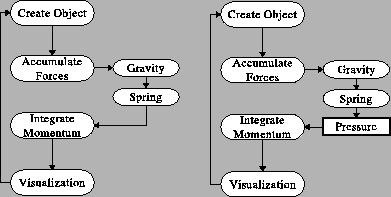Next: Create Object Up: How To Implement a Previous: Linear Spring

# Algorithm Outline

An algorithm contains 5 main steps and is exactly the same like for simple SM model. Only one difference is that we will calculate three forces instead of two which we calculate for SM. An additional Pressure Force require to calculate some additional properties of the whole body, but we will consider it later, first let us take a look on a general algorithm of our simulation program (figure 3).To show how easy an algorithm is we present comparison with an algorithm of well known SM model (left in the figure). Only one difference of Pressure Soft Body model is that we compute one additional force (pressure force). In a first step of the algorithm we init a mesh of an object. It is up to us how the mesh will be build. For our purposes we will use a parametric representation of two dimensional circle (big words for simple things :). In a second step we calculate all forces in the model. Forces will be calculated for all points on the surface. We apply three forces on the model - one external (gravity force) and two internal object forces (linear spring force and pressure force). Then simple integration of momentum equation (second Newton Law + 1st order explicit Euler integration) will be done. After those steps we will visualize our body on the screen (GLUT will be used here) and we will back to step two, where forces are calculated...

As we can see the algorithm is rather simple and our implementation will be also as simple as possible. I decided to use simlple mesh (a 2d ball), simple integration algorithm (an Euler one) and simple visualization procedures (GL Toolkit - GLUT) to make solution as simple as possible5.Next: Create Object Up: How To Implement a Previous: Linear Spring
Maciej Matyka 2004-03-30